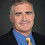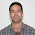## Thursday, May 05, 2016

### Open Questions

Through the years I've mentioned a few of my favorite open problems in computational complexity on this blog that have perplexed me through the years. Let me mention a few of them again in case they inspire some of the new generation of complexity theorists.
1. Does the polynomial-time hierarchy look like the arithmetic hierarchy? I mentioned this in the centenary post for Mostowski. Probably not because it would imply factoring in P (since NP∩co-NP would equal P) but we have no proof of separation and no oracle that makes them look the same.
2. Does UP = NP imply the polynomial-time hierarchy collapses? What are the consequences if SAT had an NP algorithm with a unique accepting path? Remains open, again even in relativized worlds.
3. Do rational functions that agree with Boolean functions on the hypercube have low decision tree complexity? I really expected someone to have come up with a proof or counterexample by now.
4. What happens if two queries to NP can be simulated by a single query? Does S2=ZPPNP? Both questions asked in a post on S2P.
5. Separate NP from Logarithmic space. I gave four approaches in a pre-blog 2001 survey on diagonalization (Section 3) though none have panned out. Should be much easier than separating P from NP.

1.L vs NP.
Actually, if anything ought to be "easier than P vs NP", one might as well try to look at L vs PP--one may argue that this is the biggest unknown gap among natural complexity classes. For example, if we consider the language (F,k) -- 3SAT formula F has at least k satisfying assignments--then the selfreducibilty

there is an m, such that
(F_{x1=0} , m) and (F_{x1=1} , k-m)

cannot be used by a logspace machine, as m is simply too long.
(Of course, this does not exclude potential clever algorithms that use an oracle for the j-th bit of m...)

2.What about forbidden questions? These are questions forbidden by all journals and conferences?

Rafee Kamouna.

3.I am afraid, we are flying too high, and too fast. In a danger to loose any connection to the reality. It is hard to imagine that we will solve these "big" problems without having understood "easy" ones.

My favorite example is: what is the minimal number of gates in a MONOTONE boolean circuit deciding whether a given undirected graph on {1,...,n} has a path from 1 to n. Is this number about n^3 or is smaller? That about n^3 gates are enough tells us the well-known Bellman-Ford dynamic programming algorithm.

This is a rather "pragmatic" question. Which will be not answered by any "magic" separations. So, are we on a right way by focusing on separations only?

1.'You don't get to the moon by climbing a tree.'

2.I am confused by your comment. We prove separations because we haven't been able to prove strong concrete lower bounds. Proving strong concrete lower bounds is not easier than proving separations.

4.What do you conjecture is the answer for problem 3? Is there a specific motivation for studying this problem other than it's a nice problem in decision tree complexity?

1.Problem 3 is the combinatorial analog of whether the intersection of C=P and co-C=P is equal to P. I weakly conjecture that poly-log degree rational functions that agree with a Boolean function on the hypercube has polylog decision tree complexity.

2.Thanks! Is the stronger conjecture, that P is equal to A_0PP intersect co-A_0PP (in decision tree complexity) known to be false? It sounds implausible.

5.Another approach for separating L from NP is proving branching program lower bounds:

http://dx.doi.org/10.1145/2077336.2077337

6.Has anyone ever worked on $3$?

7.As a reaction to the challenge to separate NP from Logarithmic space, I queried whether ALogTime != PH is still open (and expected to be hard to prove). The answer gave a link to a (closely related) collapse under relativization result (indicating that it is indeed still open and expected to be hard to prove).

The survey on diagonalization contains the statement that there is "no meaningful model where L and NP collapse", and the answerer admitted that he is not sure why you make that statement. Even so relativization of space bounded classes is subtle and has its quirks, the model still seems meaningful enough and allows to collapse L and NP.

1.My point with relativization is that any oracle model that allows the collapse of L and NP also allows the collapse of L and PSPACE which we know to be false in the unrelativized world.

2.Thanks for the answer. It might really help me that this is clarified, because I might want to write a survey about the connections between subroutine composition, black box oracles and white box oracles (relativization uses white box oracles) in the distant future. The second bullet point at the end of a related question "The random access to write only output memory is well outside NL. But it is still inside NP, so the composition of those NL transducers could be done inside NP. ..." might help to understand how such confusions arise and why they can be overcome.

I had the same objection as you raise in your answer, but Emil Jeřábek (May 14 '15 at 14:26) gave a good explanation how to resolve the paradox:
"Relativizing space-bounded classes is a very tricky business, and there are multiple definitions used in different contexts, but not differentiated in notation. The definition of PSPACE^{TQBF} that makes it equal to PSPACE (and to NP^{TQBF}, for that matter) is one where the oracle queries are restricted to polynomial length. The definition of PSPACE^{TQBF} for which you could relativize the space-hierarchy theorem to obtain L^{TQBF}⊊PSPACE^{TQBF} is one where oracle queries are unrestricted, and thus potentially exponentially long."

3.Let me rephrase my point. Let AP be alternating polytime (known to be PSPACE unrelativized and thus different from L). Then there is no known relativization model that both makes L = NP for some oracle but separates L from AP for all oracles.

8.Dear Professor Lance,

A major complexity class is NL. NL is the class of languages that are decidable on a nondeterministic logspace machine. A logspace machine is a Turing machine with a read-only input tape, a write-only output tape, and a read/write work tapes. The work tapes may contain at most O(log n) symbols. In addition, Sanjeev Arora described in his book "Computational complexity: A modern approach" the certificate-based definition for NL.

The certificate-based definition of NL assumes that a deterministic logspace machine verifies the elements in a NL language using another separated read-only tape for the certificate. On each step of the machine the machine's head on that tape can either stay in place or move to the right. In particular, it cannot reread any bit to the left of where the head currently is. For that reason this kind of special tape is called read once".

CLIQUE is a well-known NP-complete problem. We show that a certificate u which represents a clique V' of size k on a graph G can be verified by a deterministic logspace machine M using an additional special read-once input tape, and thereby CLIQUE will be in NL as a direct consequence of this previous definition. If any single NP-complete problem can be solved in polynomial time, then every NP problem has a polynomial time algorithm. However, every problem in NL is in P too. Consequently, we can conclude that P = NP.

You can understand more this idea and debug my verifier algorithm in

https://hal.archives-ouvertes.fr/hal-01316353/document

Any suggestion will be welcome,
1.